## Trigonometry Values Pi/9

Trigonometric functions ofradians foran integer not divisible by 3 (e.g., 40° and 80°) cannot be expressed in terms of sums, products, and finite root extractions on real rational numbers because 9 is not a product of distinct Fermat Primes. This also means that the Nonagon is not a Constructible Polygon.

However, exact expressions involving roots of complex numbers can still be derived using the trigonometric identity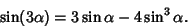(1)

Let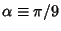and. Then the above identity gives the Cubic Equation(2)(3)

This cubic is of the form(4)

where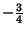(5)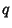(6)

The Discriminant is then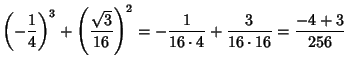(7)

There are therefore three Real distinct roots, which are approximately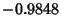, 0.3240, and 0.6428. We want the one in the first Quadrant, which is 0.3240.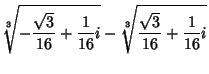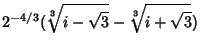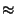(8)

Similarly,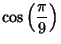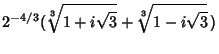(9)

Because of the Newton's Relations, we have the identities(10)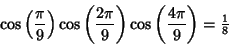(11)(12)